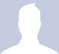## OFDM, suppressed carriers and useful data symbol rateStarted by 2 years ago2 replieslatest reply 2 years ago106 views
Hello,

I am studiyng #OFDM modulation and I think it's not quiet clear to me how to correctly set up the $$T_\text{ofdm}$$ value when we have suppressed carriers, given a certain data symbol-rate to transmit.The notations I use are:

$$r_s$$ : symbol rate.

$$T_s = r_s^{-1}$$ : symbol time.

$$N$$ : he total numbers of carriers (equivalently the FFT and IFFT coefficients).

$$N_\text{u}$$ : the carriers dedicated to symbols transmission.

I know from my professor notes that:

$$T_\text{ofdm} = N T_s \quad(1)$$

But if we have suppressed carriers, the useful data symbol-rate $$r_{s\text{u}}$$ will be:

$$r_{s\text{u}} = {N_\text{u} \over T_\text{ofdm} }$$

but since

$$N_\text{u} < N \Rightarrow r_{s\text{u}} < r_s$$

I interpret this as a loss of data.

Due to this, I would argue that to avoid this loss of data, $$T_\text{ofdm}$$ should be

$$T_\text{ofdm} = N_\text{u} T_s \quad(2)$$

The doubt comes from the fact that I know that if some symbols of a known (both from transmitter and receiver side) sequence are not transmitted, these can be recovered from the receiver, so in this case the eq. (1) is still valid, but eq.2 justifies zero-padding in frequuency domain and oversampling in time domain.

Could you clarify if my intuition of eq. 2 is definitely correct ?
Simon
[ - ]It looks to me like you're just getting tripped up on definitions and terminology, which are pretty soft, anyway.   Additionally, the time required for the cyclic prefix has been neglected, which has a pretty large affect on most of what you're looking at.

For example:

The actual length of an OFDM symbol, i.e., the repetition rate of the FFT/IFFT frames, is:

Tofdm = Tcp + Tofdm_sym

where Tcp = the length of the cyclic prefix, and Tofdm_sym is the time require to transmit the FFT/IFFT's N carriers.

To your question, all N of the FFT/IFFT samples must be transmitted, even if Nu < N, i.e., there are some suppressed subcarriers.   There are pretty much always suppressed subcarriers in order to provide guard band at the edges, and sometimes so that there is no energy at DC in the baseband signal.  Since the transform of the subcarriers is transmitted in the time domain, you must transmit all of them, populated or not.   So Tofdm_sym is proportional to N regardless of how much Nu differs from N.

So it looks to me like you're just redefining some terms to make them proportional to Nu instead of N, but that won't affect the time it takes to actually transmit a symbol.   It *will* more accurately reflect the supported data rate, since increasing the number of suppressed subcarriers reduces the occupied bandwidth and therefore the data rate.

Tcp has to be chosen based on the delay spread in the channel, so may differ significantly between applications, but may be as much as Tofdm_sym/3.

[ - ]